# Overview

Population time plots can be extremely informative graphical displays of survival data. They should be the first step in your exploratory data analyses. We facilitate this task in the casebase package by providing a plot method for objects returned by the popTime function. This is done in two steps:

1. Pass your dataset to the casebase::popTime function and specify the column names corresponding to
• the time of event
• the event status
• exposure (optional)

This will create an object of class popTime with an exposure attribute if you specify a value for the exposure argument.

1. Pass the object of class popTime to the plot function

In this vignette we show how to create population-time plots for the two datasets that ship with the casebase package, as well as several well known survival datasets from the survival package. For a more detailed description of how to modify the aesthetics of a population time plot, please refer to the Customizing Population Time Plots vignette.

library(survival)
library(casebase)
library(ggplot2)
library(data.table)

# European Randomized Study of Prostate Cancer Screening Data

For our first example, we make use of the European Randomized Study of Prostate Cancer Screening (ERSPC) data which ships with the casebase package (see help("ERSPC", package = "casebase") for details about this data).

data("ERSPC")
head(ERSPC)
##   ScrArm Follow.Up.Time DeadOfPrCa
## 1      1         0.0027          0
## 2      1         0.0027          0
## 3      1         0.0027          0
## 4      0         0.0027          0
## 5      0         0.0027          0
## 6      0         0.0027          0

We first pass this dataset to the popTime function. If you do not specify the time and event arguments, this function will try to guess them using regular expressions. See the Details section of help("popTime", package = "casebase") for how we try to guess these inputs.

pt_object <- casebase::popTime(ERSPC, event = "DeadOfPrCa")
## 'Follow.Up.Time' will be used as the time variable

We can see its contents and its class:

head(pt_object)
##    ScrArm   time event original.time original.event event status ycoord yc
## 1:      1 0.0027     0        0.0027              0     censored 159893  0
## 2:      1 0.0027     0        0.0027              0     censored 159892  0
## 3:      1 0.0027     0        0.0027              0     censored 159891  0
## 4:      0 0.0027     0        0.0027              0     censored 159890  0
## 5:      0 0.0027     0        0.0027              0     censored 159889  0
## 6:      0 0.0027     0        0.0027              0     censored 159888  0
##    n_available
## 1:           0
## 2:           0
## 3:           0
## 4:           0
## 5:           0
## 6:           0
class(pt_object)
##  "popTime"    "data.table" "data.frame"

The casebase package has a plot method for objects of class popTime:

# plot method for objects of class 'popTime'
plot(pt_object)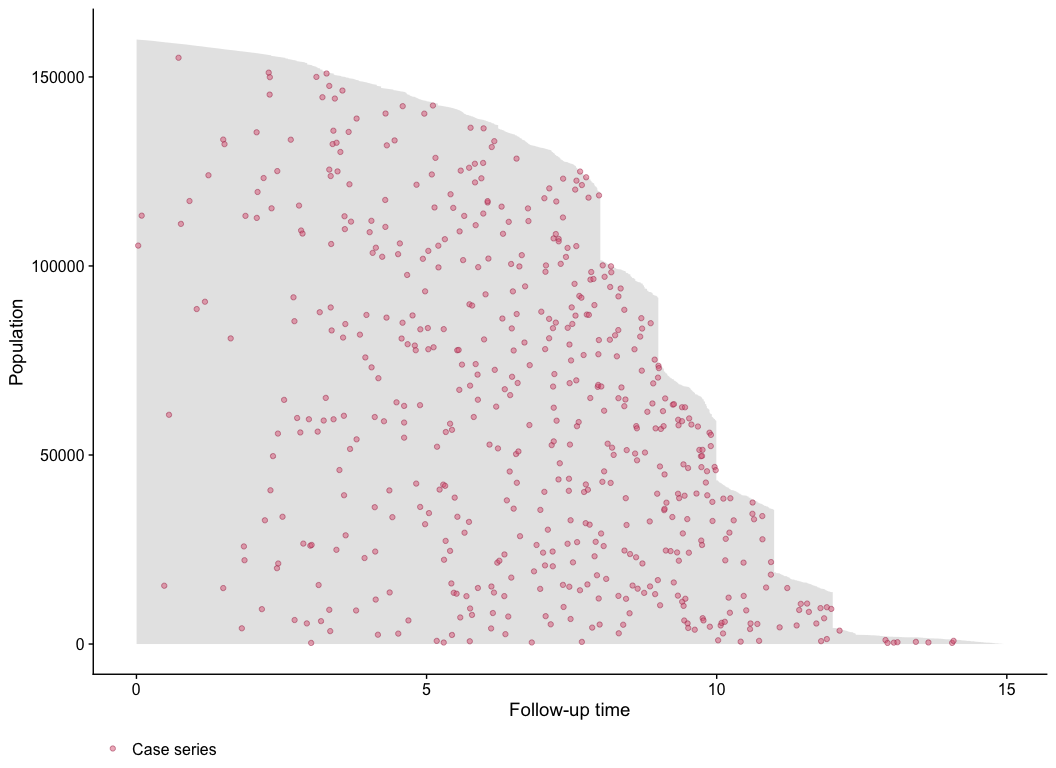One benefit from these plots is that it allows you to see the incidence density. This can be seen from the distribution of the red dots in the above plot. We can see that more events are observed later on in time. Therefore a constant hazard model would not be appropriate in this instance as it would overestimate the cumulative incidence earlier on in time, and underestimate it later on.

The unique ‘step shape’ of the population time plot is due to the randomization of the Finnish cohorts which were carried out on January 1 of each of the 4 years 1996 to 1999. This, coupled with the uniform December 31 2006 censoring date, lead to large numbers of men with exactly 11, 10, 9 or 8 years of follow-up.

It is important to note that the red points are random distributed across the gray area for each time of event. That is, imagine we draw a vertical line at a specific event time. We then plot the red point at a randomly sampled y-coordinate along this vertical line. This is done to avoid having all red points along the upper edge of the plot (because the subjects with the least amount of observation time are plotted at the top of the y-axis). By randomly distributing them, we can get a better sense of the incidence density.

## Exposure Stratified Population Time Plot

Often the observations in a study are in specific groups such as treatment arms. It may be of interest to compare the population time plots between these two groups. Here we compare the control group and the screening group. We create exposure stratified plots by specifying the exposure argument in the popTime function:

# Appropriately label the factor variable so that these labels appear in the
# stratified population time plot
ERSPC$ScrArm <- factor(ERSPC$ScrArm,
levels = c(0,1),
labels = c("Control group", "Screening group"))
pt_object_strat <- casebase::popTime(ERSPC,
exposure = "ScrArm")
## 'Follow.Up.Time' will be used as the time variable

We can see its contents, class and that it has an exposure attribute:

head(pt_object_strat)
##           ScrArm   time event original.time original.event event status ycoord
## 1: Control group 0.0027     0        0.0027              0     censored  88232
## 2: Control group 0.0027     0        0.0027              0     censored  88231
## 3: Control group 0.0027     0        0.0027              0     censored  88230
## 4: Control group 0.0027     0        0.0027              0     censored  88229
## 5: Control group 0.0137     0        0.0137              0     censored  88228
## 6: Control group 0.0137     0        0.0137              0     censored  88227
##    yc n_available
## 1:  0           0
## 2:  0           0
## 3:  0           0
## 4:  0           0
## 5:  0           0
## 6:  0           0
class(pt_object_strat)
##  "popTime"    "data.table" "data.frame"

We can also see that the pt_object_strat has an exposure attribute which contains the name of the exposure variable in the dataset:

attr(pt_object_strat, "exposure")
##  "ScrArm"

The plot method for objects of class popTime will use this exposure attribute to create exposure stratified population time plots:

plot(pt_object_strat)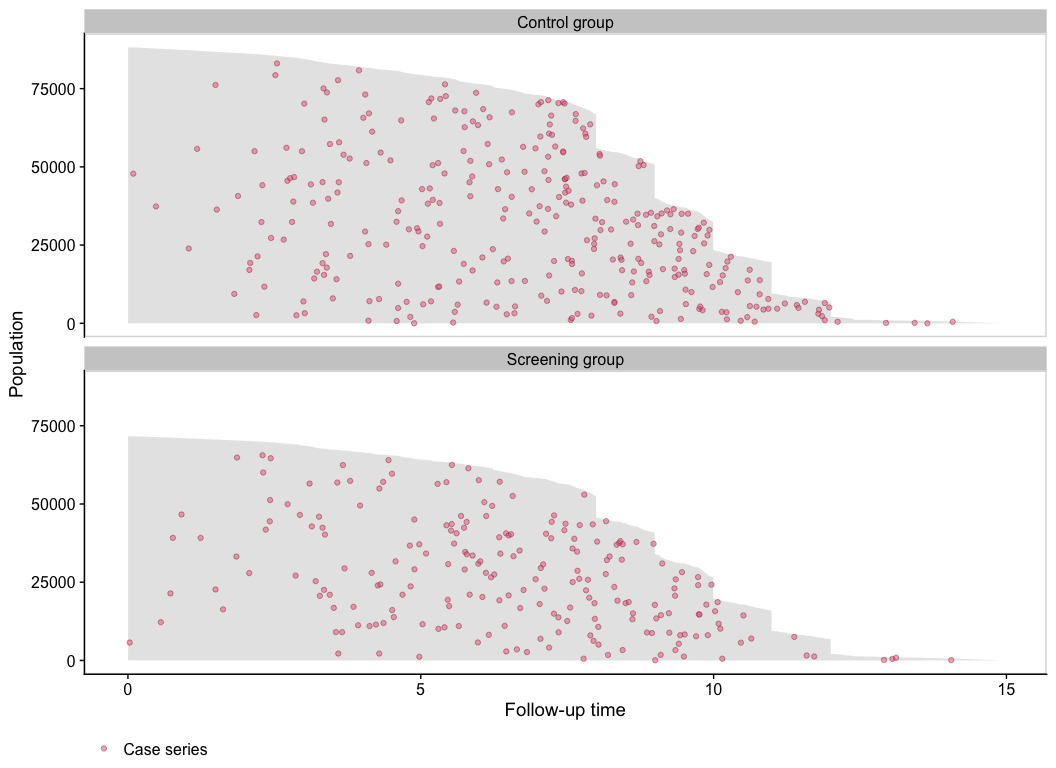We can also plot them side-by-side using the facet.params argument, which is a list of arguments that are passed to the facet_wrap() function in the ggplot2 package:

plot(pt_object_strat,
facet.params = list(ncol = 2))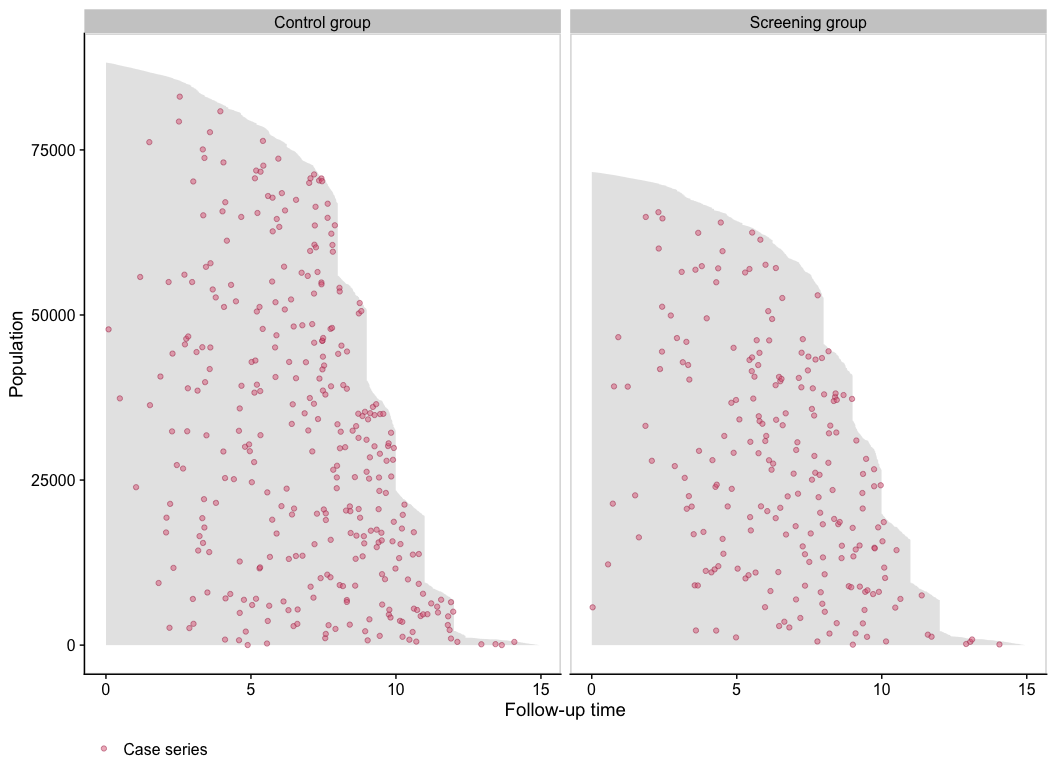## Plotting the base series

To illustrate the casebase sampling methodology, we can also plot the base series using the add.base.series function:

plot(pt_object_strat,
add.base.series = TRUE)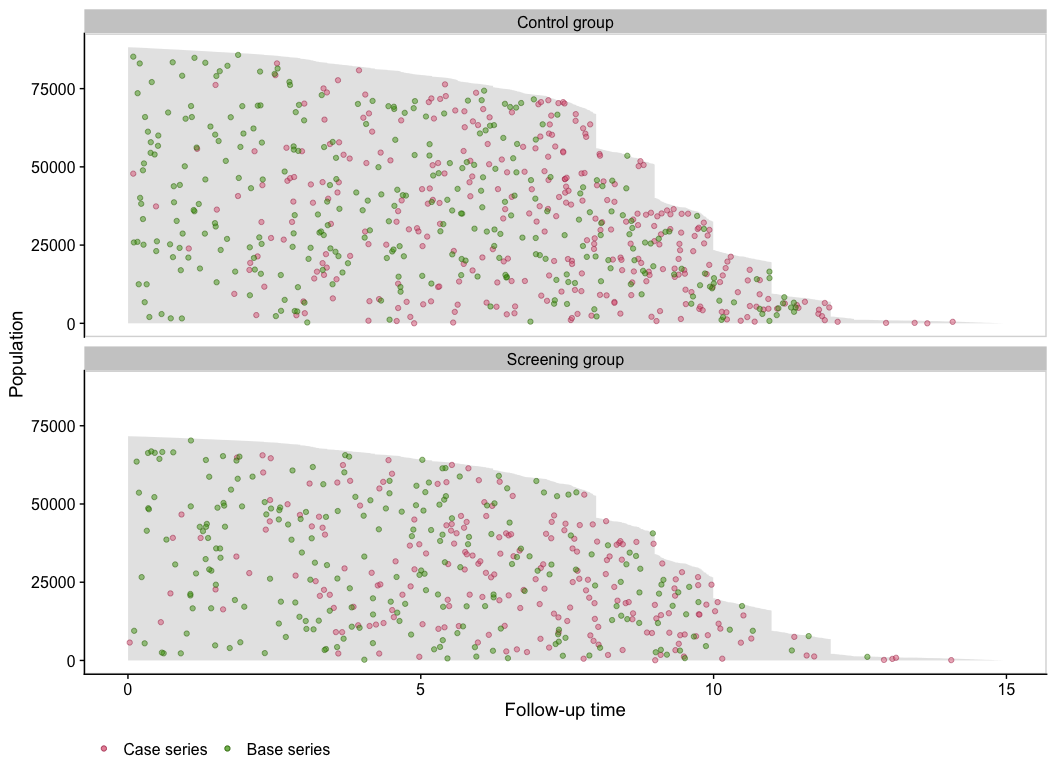Note that the theme.params argument is a list of arguments passed to the ggplot2::theme() function.

# Stem Cell Data

Next we show population time plots when there is a competing event. The bmtcrr dataset contains information on patients who underwent haematopoietic stem cell transplantation for acute leukaemia. This data is included in the casebase package. See help("bmtcrr", package = "casebase") for more details. We will use this dataset to further illustrate the fundamental components of a population time plot.

Note that for this dataset, the popTime fails to identify a time variable if you didn’t specify one:

# load data
data(bmtcrr)
str(bmtcrr)
## 'data.frame':    177 obs. of  7 variables:
##  $Sex : Factor w/ 2 levels "F","M": 2 1 2 1 1 2 2 1 2 1 ... ##$ D     : Factor w/ 2 levels "ALL","AML": 1 2 1 1 1 1 1 1 1 1 ...
##  $Phase : Factor w/ 4 levels "CR1","CR2","CR3",..: 4 2 3 2 2 4 1 1 1 4 ... ##$ Age   : int  48 23 7 26 36 17 7 17 26 8 ...
##  $Status: int 2 1 0 2 2 2 0 2 0 1 ... ##$ Source: Factor w/ 2 levels "BM+PB","PB": 1 1 1 1 1 1 1 1 1 1 ...
##  $ftime : num 0.67 9.5 131.77 24.03 1.47 ... # table of event status by exposure table(bmtcrr$Status, bmtcrr\$D)
##
##     ALL AML
##   0  17  29
##   1  28  28
##   2  28  47
# error because it can't determine a time variable
popTimeData <- popTime(data = bmtcrr)
## Error in checkArgsTimeEvent(data = data, time = time, event = event): data does not contain time variable

In this case, you must be explicit about what the time variable is:

popTimeData <- popTime(data = bmtcrr, time = "ftime")
## 'Status' will be used as the event variable
class(popTimeData)
##  "popTime"    "data.table" "data.frame"

## Plotting the follow-up times for each observation

We first plot that area on the graph representing the observed follow-up time. Fundamentally, this area is constructed by plotting a line for each individual, where the length of each line represents their follow-up time in the cohort. The follow-up times are plotted from top (shortest follow-up time) to bottom (longest follow-up time). In practice, we instead plot a polygon using the ggplot2::geom_ribbon() function. The following figure shows this area for the bmtcrr dataset. Note that we must specify add.case.series = FALSE because the default is to add the case series:

plot(popTimeData,
add.case.series = FALSE)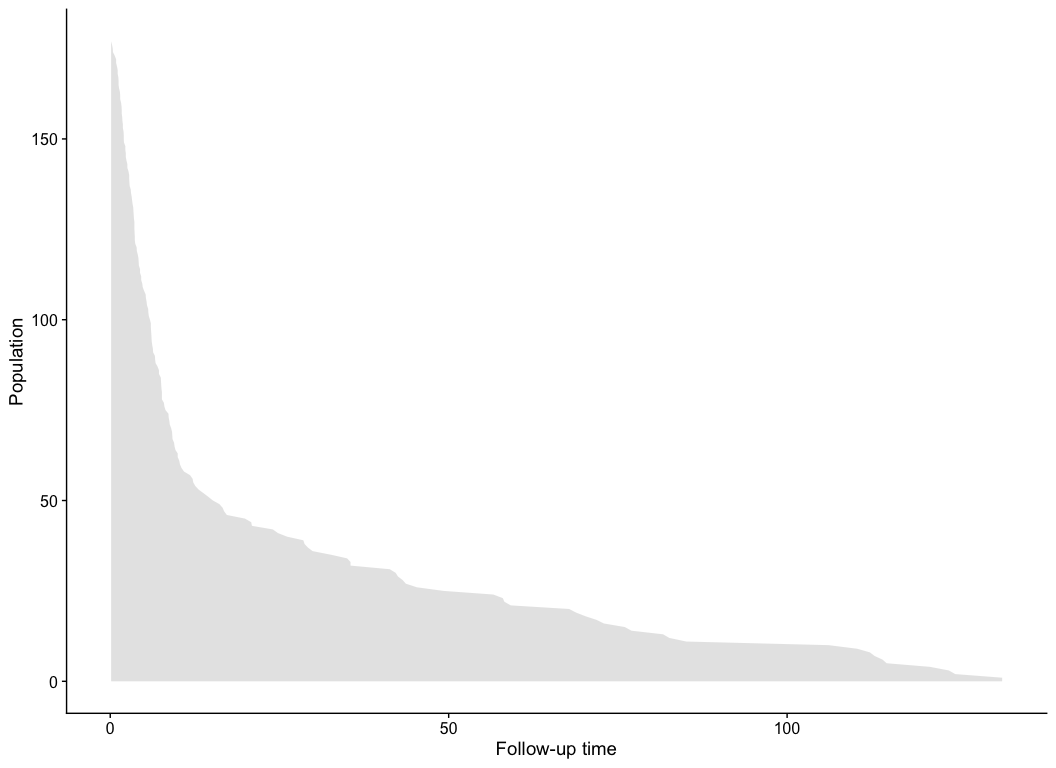Note that we can change the aesthetics of the area by using the ribbon.params() argument as follows. These arguments are passed to the ggplot2::geom_ribbon() function:

plot(popTimeData,
ribbon.params = list(color = "red", fill = "blue", size = 2, alpha = 0.2))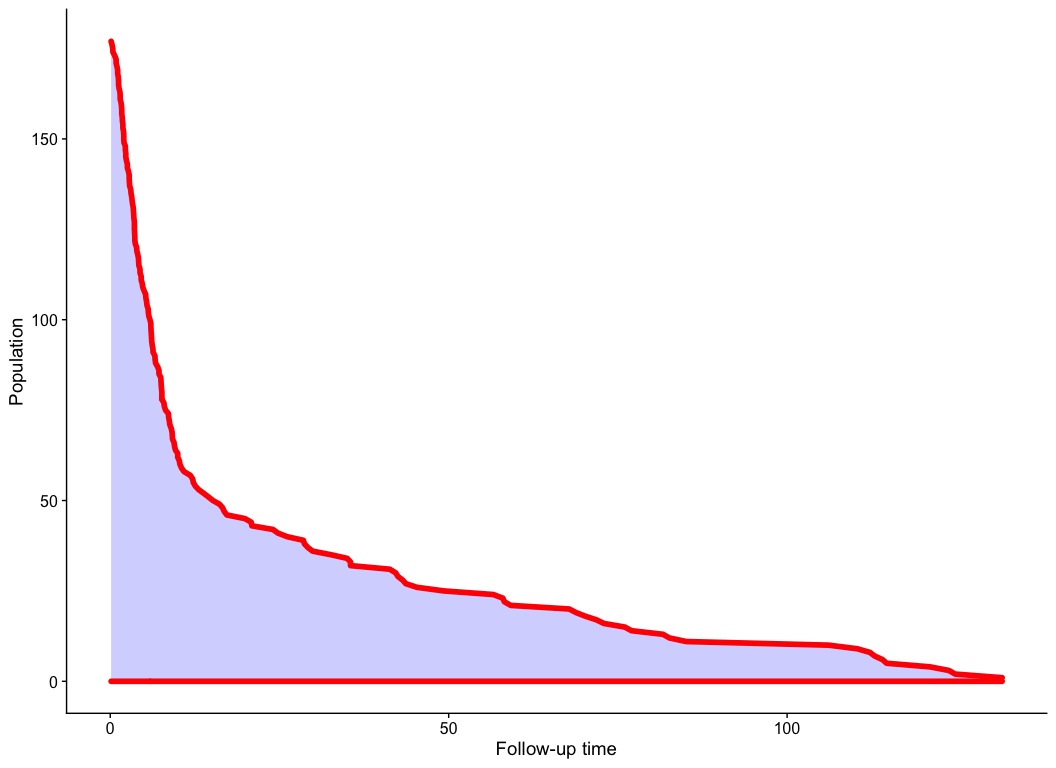## Plot the Case Series

Next we add the case series. Note that because the Status column has a competing event (coded as 2), we must specify comprisk = TRUE (even if you don’t want to plot the competing event):

plot(popTimeData,
comprisk = TRUE)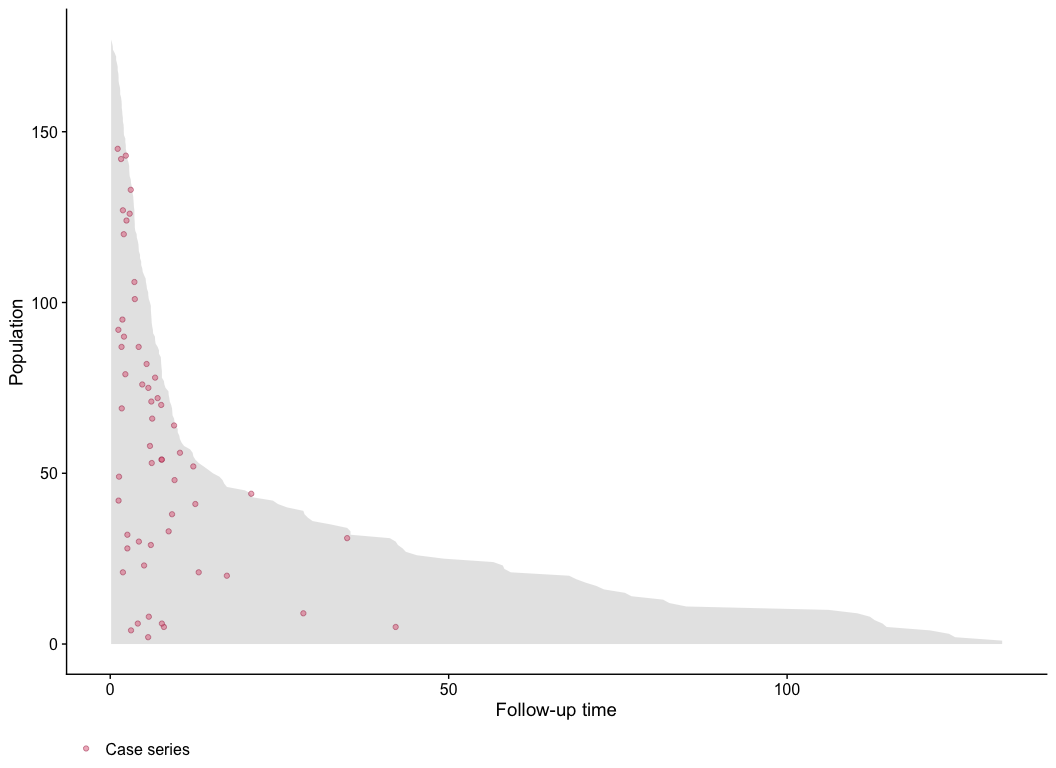In the above plot we can clearly see many of the deaths occur at the beginning, so in this case, a constant hazard assumption isn’t valid. This information is useful when deciding on the type of model to use.

## Plot the Base Series

We can now add the base series with the add.base.series argument. Internally, the plot method calls the casebase::sampleCaseBase function to sample the base series from the total person moments. This requires us to specify the ratio of base series to case series in the ratio argument which we will leave at its default of 1. A legend is also added by default:

plot(popTimeData,
comprisk = TRUE)